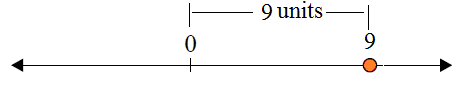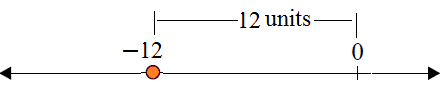# Absolute value of a number

The marks || (parallel bars) around a number give the absolute value of the number.

The absolute value of a number is its distance from 0 on the number line.

Also, the absolute value of any number (other than 0) is always positive.

### Formula

|a|, the absolute value of ‘a’, is its distance ‘a’ units from 0 on the number line.

For example

|9|, the absolute value of ‘9’, is its distance ‘9’ units from 0 on the number lineSimilarly,

|−12|, the absolute value of ‘−12’, is its distance ‘12’ units from 0 on the number lineFind the absolute value of the following number:

35

### Solution

Step 1:

The absolute value of any number (other than 0) is always positive.

Step 2:

The absolute value of the number 35 is

|35| = 35

Find the absolute value of the following number:

−72

### Solution

Step 1:

The absolute value of any number (other than 0) is always positive.

Step 2:

The absolute value of the number −72 is |−72| = 72

Find the following absolute value:

|9−2|

### Solution

Step 1:

The absolute value of any number (other than 0) is always positive.

Step 2:

The absolute value is found by simplifying.

|9−2| = |7| = 7